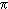# 6

This number is a composite.The first pair of consecutive primes that differ by 6 are 23 and 29.6 is the smallest number which is the product of two distinct primes. [Brueggeman]The only mean between a pair of twin primes which is triangular. [Shanks]The probability that a number picked at random from the set of integers will have no repeated prime divisors is 6/π2. [Chartres]Was Robert Breusch the first to prove that there exists at least one prime of the form 4k + 1 and at least one prime of the form 4k + 3 between n and 2n for all n greater than 6? See Mathematische Zeitschrift.Prime doubles of the form (p, p + 6) are called sexy primes.± 1 + 234 * 56 are twin primes. [Kulsha]The product of the first n Mersenne prime exponents + 1 is prime for n = 1, 2, 3, 4, 5 and 6.If p ± 1 is a twin prime greater than 6 then p = 6n, where n is a positive integer. [Goldstein]6 is the smallest composite number whose sum of prime factors is prime. [Gupta]The smallest invertible brilliant number. [Capelle]6 is the only number such that the sum of all the primes up to 6 equals the sum of all the composite numbers up to 6. [Murthy]6*66*666*6666*66666*666666 + 1 is prime. [Poo Sung]The only perfect number that can be sandwiched between twin primes. [Gupta]6 is the smallest composite number such that the concatenation (23) as well as the sum (5) of its prime factors are prime. [De Geest]It is a theorem that primes of form 6n ± 1 oscillate largest numbers an infinite number of times. [Ribenboim]The proper factors of 6 are 1, 2 and 3. Note that 2*6^3-1, 2*6^3+1, 3*6^2-1 and 3*6^2+1 are all prime numbers. [Hartley]6 + 1, 6 + 66 + 1, 6*66 + 1 , 6 + 66 + 666 + 1 and 6*66*666 + 1 are all primes. [Firoozbakht]The product of the first four nonzero Fibonacci numbers. Note that 6 + 1 and 6 - 1 are twin primes. This is the smallest such product of consecutive nonzero Fibonacci numbers. [Gupta]6 is the smallest value for n such that n-1, n+n^2-1, n+n^2+n^3-1, and n+n^2+n^3+n^4-1 are all primes. [Opao](π(6)*6*6 - 1, π(6)*6*6 + 1 ) are twin primes. [Firoozbakht]All numbers between twin primes are evenly divisible by 6. [Smith]For n = 1, 2, 3, 4, 5^n + 6 is prime. [Rosenfeld]The lowest composite number containing distinct prime factors that cannot be expressed as the sum of two distinct prime numbers. [Capelle]The only integer which admits strictly fewer prime compositions (ordered partitions) than its predecessor. [Rupinski]The smallest invertible semiprime. [Capelle]The only semiprime whose divisors are consecutive numbers. [Silva]The smallest number whose factorial is a product of two factorials of primes (6! = 3!5!). Note that 6 is one of these factorials, in accordance with the equation (n!)! = n!(n! - 1)! [Capelle]F0*F1*F2*...*F6*(F6 - 1) - 1 is prime, where Fn denotes the nth Fermat number. [Wesolowski]The number of prime Euler's "numeri idonei". [Capelle]6 is probably the only even number n such that both numbers n^n - (n+1) and n^n + (n+1) are primes. [Firoozbakht](1# + 2# + 3# + 4# + 5# + 6#) is one-half of(1! + 2! + 3! + 4! + 5! + 6!). [Wesolowski]The first perfect number.6 + 66 + 666 + 6666 + 66666 + 666666 + 1 is prime. [Homewood]6 is the only perfect number that is a semiprime. [King]There are only six prime-digit primes with distinct odd digits, i.e., 3, 5, 7, 37, 53, 73. [Loungrides]6^6 + 5^5 + 4^4 + 3^3 + 2^2 + 1^1 is prime. [Meyers]The smallest number of primes that can be found on the Tetractys Puzzle. [Keith]6 is the product of the first two primes. [Jeursen]The only perfect oblong number, i.e., 6=2*3. [Loungrides]The start of a sequence of invertible semiprimes (numbers when inverted give a different semiprime): 6, 9, 106, 119, 611, 901, ... . Can you find the first case where both reversals are also different semiprimes? Dare we call them "bemirpimes" (short for bi-directional emirpimes)?The last digit to appear in a Sophie Germain prime, first appearing in 641. The other 9 digits each first appear in smaller Sophie Germain primes. [Gaydos]There are 6 primes that can increase your conversion.In mathematics, 6 is considered to be the first ‘perfect’ number. [Oliveira]6 is the smallest semiprime for which order there is no field. [Pankajjyoti]6 is the smallest composite number whose prime factors contain distinct digits exactly once each:
The finite sequence of such numbers start as 6, 10, 14, 15, 21, 26, 30, 34, 35, 38, 42, 51, 57, 62, 65, 70, 74, 82, 85, 86, 87, 91, 94, 95, 102, 105, 106, 114, 115, 118, 122, 123, 130, 133, 134, 141, 142, 145, 146, 155, 158, 161, 166, 170, 177, 178, 182, 183, 185, 190, 194, 201, 203, 205, 206, 210, 213, 214, 215, 217, 218, 235, 237, 246, 255, 266, 267, 274, 278, 282, 285, 287, 291, 298, 301, 305, 310, 314, 321, 326, 327, 334, 335, 346, 354, 355, 358, 365, 366, 370, 371, 377, 381, 386, 391, 394, 395, 399, 402, 410, 413, 415, 426, 427, 430, 434, 435, 437, 445, 447, 455, 470, 471, 474, 485, 493, 501, 510, 515, 534, 535, 537, 545, 570, 574, 581, 582, 591, 602, 609, 610, 611, 614, 615, 623, 634, 635, 642, 654, 665, 670, 685, 694, 695, 698, 703, 705, 710, 718, 721, 723, 730, 731, 734, 742, 745, 753, 758, 763, 767, 771, 778, 790, 794, 798, 802, 805, 807, 813, 815, 817, 818, 826, 830, 835, 838, 843, 854, 861, 862, 865, 871, 878, 890, 893, 894, 895, 899, 901, 910, 914, 915, 922, 926, 934, 942, 943, 958, 965, 970, 973, 974, 982, 985, 1002, ..., with 8439563243 being the largest. How many terms are there? [Honaker]

(There are 3 curios for this number that have not yet been approved by an editor.)